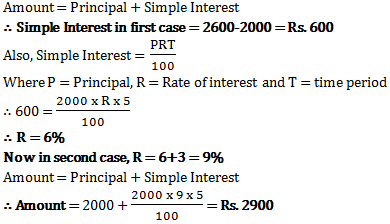# Simple Interest - Quantitative Aptitude (MCQ) questions for Q. 29458

Q.  Ram gets Rs. 2600 for Rs. 2000 in 5 years at some rate of simple interest. Had he invested in other places where rate of simple interest is 3% more than current rate, how much would have Ram got in same time?
- Published on 13 Apr 17

a. Rs. 2900
b. Rs. 3000
c. Rs. 3100
d. Rs. 2800